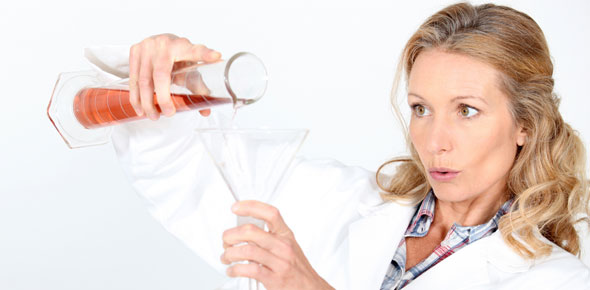# Chapter 6 - Chapter Test (10 Questions)

12 Questions | Attempts: 113SettingsChapter 6, Reference: Prentice Hall, publication 2004.

• 1.
What is an indicator used for in science?
• A.

Find the "pH" of a solution (tells how much "potential of Hydrogen" is in it)

• B.

Find the "concentration" of a solution (amount of one material dissolved in another)

• C.

Test for "conductivity" (if it allows electricity to flow)

• D.

Find out if a solution is "saturated" (full to the most amount of solute can hold)

• 2.
What type of solution has the pH of 9?
• A.

Acidic

• B.

Neutral

• C.

Basic

• D.

Saturatede

• 3.
What is a "characteristic property" of acids?
• A.

Acids turn red litmus paper "blue"

• B.

Acids taste bitter

• C.

Acids turn blue litmus paper "red"

• D.

Acids "do not" react with metals

• 4.
What is a liquid mixture in which particles "can be seen" and "easily separated by filteration"?
• A.

Solution

• B.

Suspension

• C.

Solvent

• D.

Colloid

• 5.
When 40 mL of alcohol form a solution with 30 mL of water***"Think how much water and how much alcohol. Then think which is the solvent (large amount) or solute (smallest amount).
• A.

Alcohol is the solvent

• B.

Water is the solvent

• C.

Both alcohol and water are solvents

• D.

Neither alcohol nor water is a solvent

• 6.
How can a scientist tell whether a water solution contains salt of sugar?
• A.

By tasting the solution

• B.

By smelling the solution

• C.

By testing the electrical conductivity of the solution

• D.

By filtering the solution

• 7.
When a compound dissolves in water
• A.

It always breaks up into ions (electrically charged atoms)

• B.

Its always conducts electricity

• C.

Its particles surround individual warter molecules

• D.

Each of its particles is surrounded by water molecules

• 8.
Weak tea is an example of a
• A.

Dilute solution

• B.

Concentrated solution

• C.

Saturated solution

• D.

Supersaturated solution

• 9.
What do bases form in water?
• A.

Hydroxide ions (H0-)?

• B.

Oxide ions

• C.

Hydrogen ions (H+)

• D.

Gas

• 10.
What is the pH of normal rainfall which is slightly acidic?
• A.

1

• B.

Less than 3

• C.

Between 5 and 7

• D.

Between 10 and 12

• 11.
What is the reaction between an acid and a base?
• A.

Neutralization

• B.

Action-reaction

• C.

Basicity

• D.

Chemical reaction

• 12.
Which is an example of a chemical change?
• A.

Grating cheese

• B.

Ripping paper

• C.

Digestion

• D.

Sawing a piece of wood apart

## Related TopicsBack to top
×

Wait!
Here's an interesting quiz for you.